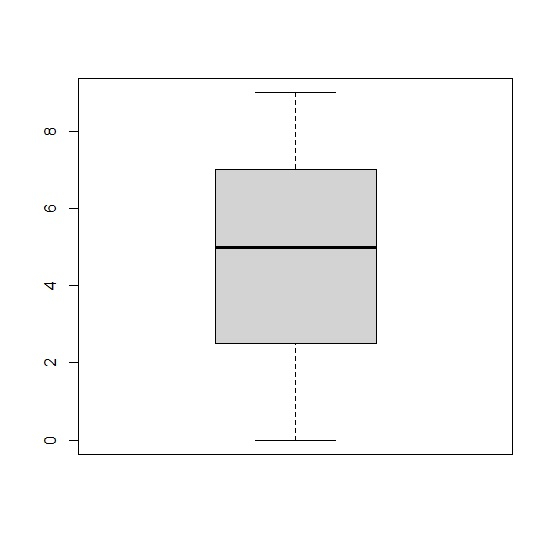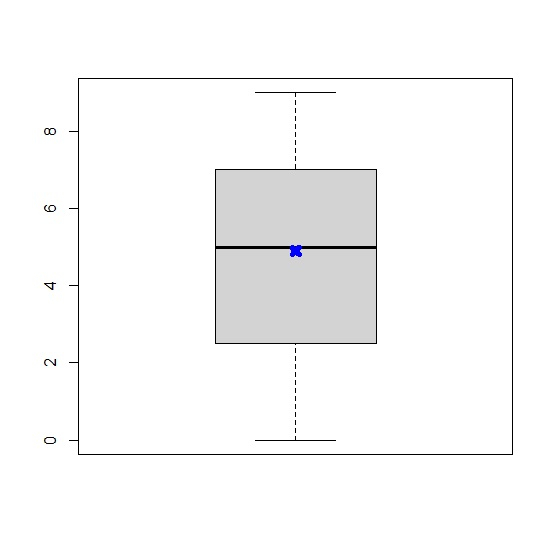# How to display mean in a boxplot with cross sign in base R?

To display mean in a boxplot with cross sign in base R, we can use the points function and pass the mean with pch = 4 that represents a star, also we can change the color to highlight the mean using col argument and the size of the start can be changed using lwd argument as shown in the below examples.

## Example

Live Demo

x<-sample(0:9,100,replace=TRUE)
boxplot(x)

## Output## Example

points(mean(x),col="blue",pch=4,lwd=6)

## Output